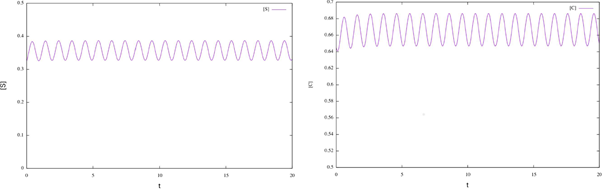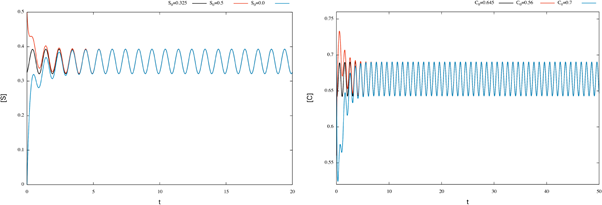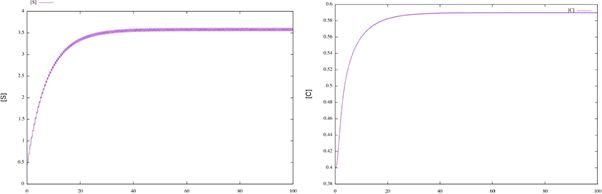## Artigo

•Citado por SciELO
•Acessos

•Citado por Google
•Similares em SciELO
•Similares em Google

## versão impressa ISSN 0120-419X

### Integración - UIS vol.37 no.2 Bucaramanga jul./dez. 2019  Epub 10-Out-2019

#### http://dx.doi.org/10.18273/revint.v37n2-2019005

Artículos originales

Oscillations in enzymatic reactions with periodic input

Oscilaciones en reacciones enzimáticas con entradas periódicas

Brenda Lara-Agüilara  , Osvaldo Osunaa  , Giovanni Wencesb

a Universidad Michoacana de San Nicolás de Hidalgo, Instituto de Física y Matemáticas, Michoacán, México.

b Universidad Autónoma de Guerrero, Escuela Superior de Matemáticas Núm. 2, Guerrero, México.

Abstract

In this work, we prove the existence of periodic solutions for some enzyme catalyzed reaction models subject to periodic substrate input. We also obtain uniqueness and asymptotic stability of the periodic solution of some classes of reaction equations. Numerical simulations are performed using specific substrate functions to illustrate our analytical findings.

MSC2010: 34C25, 92C45, 47H1.

Key words: Cooperative systems; enzyme kinetics; periodic orbits

Resumen

En este trabajo probamos la existencia de soluciones periódicas para algunos modelos de reacciones catalizadas por enzimas sujetas a una entrada periódica de sustrato. También obtenemos unicidad y estabilidad asintótica de la solución periódica de algunas clases de reacciones. Realizamos simulaciones numéricas utilizando funciones específicas de sustrato para ilustrar nuestros hallazgos analíticos.

Palabras-clave: Sistemas cooperativos; cinética enzimática; órbitas periódicas

1. Introduction

Mathematical models have become important tools in analyzing chemical reactions; of special interest are enzymatic reactions in biochemical systems. Enzymes are important in regulating biological processes acting as activators or inhibitors in a reaction. A basic scheme for a catalyzed reaction is based into the Michaelis-Menten equation, which is fundamental for enzyme kinetics. It can be described by the following kinetic mechanism:

where E denotes enzyme, S denotes substrate, P denotes product and C denotes enzyme substrate complex. I(t) is the rate of input of the substrate into the system. Letting [A] denote concentration of a chemical specie A. A well-studied law of action kinetic is the law of mass action; it assumes that the rate of a reaction is proportional to the concentrations of its reactants; the law of mass action leads to the system of following non-linear reaction equations:

All of the parameters are positive constants. Since the scheme (1) is reversible, then, by adding the second and third equations in the system (2), we have ([E] + [C])’ = 0; therefore [E] + [C] = K for all time t for some positive constant K. Using this relation, and since the last equation in system (2) decouples from the other equations, we can reduce model (2) to a two-dimensional system in terms of [S] and [C], as follows:

In chemical engineering it is common to use stirred tank reactors where fresh nutrient are supplied at periodic rate from external sources; on the other hand, a natural situation in biological system is that the rate of input of the substrate I fluctuates in periodic form; this is due to intrinsic oscillations in living organisms. So, for the interest of the chemical industry or for biological reasons of the reactions considered, we can assume that I is a non negative, non constant continuous I-periodic function;

In the study of enzymatic models the analysis of periodic solutions is seen as an important goal, since this periodicity reveals the recurrence of biochemical rhythms of living organisms. Hence, determining existence of such solutions under different parameter configurations and input functions is crucial.

Stoleriu and coworkers  studied the existence of periodic solutions in a particular class of input rate I, by using Brouwer's fixed-point theorem. Katriel  proved the existence of periodic orbits of (3), by using Leray-Schauder degree theory. Moreover, he asked if that periodic solution is unique and asymptotically stable. Using the theory of cooperative systems, in the sequel we will recover Katriel's result as an application of our main result; moreover, we obtain the uniqueness of such orbit, and that the periodic solution attracts all other positive solutions, answering affirmatively to Katriel's questions . On the other hand, considering the generalized mass-action law we obtain more general systems of type (3); for that kind of systems we also prove the existence of periodic orbits.

2. Results

We first review the cooperative systems (this material is included for completeness and to fix some of the notation); for a brief introduction to cooperative systems see . For two points x, y ∈ ℝn denote the partial order u ≤ v, if ui vi for each i; also denote u < v if u v and u ≠ v. Consider a system

where f,g are C1 in an open D ⊂ ℝ2 and continuous T-periodic functions on t. Recall that (4) is said a cooperative system in ℝ x D if

The cooperative systems have very important properties. For example: the monotonicity of the local flow generated by (4).

We say that a pair of T-periodic differentiable functions (a(t),b(t)) is a subsolution pair of (4) if

Analogously, a pair of T-periodic differentiable functions (A(t), B(t)) is a supersolution pair if

We say that sub-and supersolution pairs are ordered if for all t we have a(t) < A(t) and

Finally, we say that a solution (u(t), w(t)) of (4) is globally attracting on a positively invariant set R ⊆ ℝ2 if all solutions (x(t),y(t)) with (x(0),y(0)) ∈ R satisfy

An important feature for the cooperative system (4) about periodic orbits was established in , Theorem 2.1. More precisely, the following result holds.

Theorem 2.1. Assume that the system (4) is cooperative and has ordered sub- and super-solution pairs (a(t),b(t)) and (A(t), B(t)). Then the system has a T-periodic solution, satisfying a(t) < x(t) < A(t),b(t) < x(t) < B(t),t. Furthermore, any solution of (4), with the initial (x(0), y(0)) ∈ (a(0),A(0)) x (b(0), B(0)), converges to the productwhereare the minimal, maximal T-periodic solution, respectively.

Now we state our first result

Theorem 2.2. Assume k 1 , k 2 , k -1 , K > 0, and I(t) is a non negative, non constant continuous T-periodic function. Then we get:

i) There is at least one T-periodic solution [S(t)], [C(t)] of (3) whose components are positive if, and only if,

ii) Under (8) the T-periodic solution is unique in

iii) It attracts all other positive solutions of (3), when t → ∞.

Proof. i) It is clear that (8) is a necessary condition for the existence of a T-periodic solution. In fact by adding both equations in (3) and integrating the result on [0,T]. Now assume the condition (8); first, note that system (3) is of cooperating type, so to use Theorem 2.1 we need construct sub- and super-solution pairs. First we take (a(t), b(t)) = (0, 0), which is a subsolution pair.

For the super-solution pair we take A(t) to be the unique positive T-periodic solution of

with θ and M < K positive constants to be fixed later. Also we take B(t) = M. To obtain that (A(t), B(t)) are a supersolution pair, it must be fulfilled that

And

Now, the periodic solutions A(t) of (9) (as function of M) satisfy

Taking the limit M → K in the right side of (11) we get which is negative by (8) for θ sufficiently small; therefore, the inequality (11) is satisfied for θ small and M close to K. Thus we have sub- and super-solution pairs; then, by Theorem 2.1. there is at least one T-periodic solution, which proves i).

ii) By adding both equations in (3) and integrating for a periodic solution we get

this is also true for the maximal periodic solution i.e.,

thus, from (12) and (13) we have

therefore for any periodic solution we have but from the second equation in (3) we obtain that this establishes the uniqueness.

iii) Note that the band (0,K) is attractor to (3) in ℝ2, and by Theorem 2.1 there is a unique periodic solution in (0,A(0)) x (0,K) which is attractor in this set, where A is the unique periodic solution of (9). Since

then A(0) becomes arbitrarily large. Then the periodic solution is globally attracting on all positive solutions of (3), when t → ∞

As we have really mentioned Katriel  proved the existence of periodic orbits of (3) under the condition (8), by using Leray-Schauder degree theory. Moreover, he proposed the next question: is it true that if the periodic solution is unique and globally stable? From our result we obtain a different proof of existence, and we answer affirmatively his questions.

It should be noted that the existence of periodic orbits in the particular case of I(t): = I 0 (1 + є sin(wt)) was considered in  by using Brouwer's fixed-point theorem. Observe that Theorem 2.2 gives an alternative proof to Theorem 1 in , which we remember below.

Corollary 2.3. Let I(t): = I 0 (1 + є sin(wt)), with 0 ≤ є ≤ 1. If

then for any value of є, 0 ≤ є ≤ 1, the system (3) has at least one positive-periodic solution. Moreover, the periodic solution /or e sufficiently small is unique.

Note that the condition (15) implies (8), but it is a stronger condition; moreover, we do not require additional conditions for uniqueness (over є), therefore Theorem 2.2 produces a slight generalization of this result.

To model the dynamics of the concentrations of the chemical species involved, we also can consider the generalized mass-action law (see ). Under this law we obtain a more general enzyme catalysed system than (3), written as

All of the parameters (exponents included) are positive constants and I is a non constant continuous T-periodic function.

With a similar proof as in Theorem 2.2, we obtain the following slight generalization of this result:

Theorem 2.4. Assume k 1 , k 2 , k -1 , K > 0, and I(t) is a non negative, non constant continuous T -periodic function. If β > 0 and α = 1, then we get:

i) There is at least one T-periodic solution [S(t)], [C(t)] of (16) whose components are positive if, and only if, (8) holds.

ii) Under (8) the T-periodic solution is unique in

iii) It attracts all other positive solutions of (16), as t → ∞.

2.1. Examples

In the previous section we established analytically the existence of periodic solutions for system (2) of enzymatic reactions. The object of this section is to show numerical evidence of the existence of periodic solutions. We numerically solved these equations using a 4th order accurate Runge-Kutta integrator, which was programmed in FORTRAN 95. The graphs correspond to numerical approximations of the periodic analytic solution; the parameters are artificial, and only for the purpose of illustrating our analytical results.

Example 1. Consider the system

where parameters are determined by k 1 = 2, k -1 = 1, k 2 = 1.5, and K = 33. The substrate input function is determined by I(t) = 1 + 0.3 sin(2πt). Note that 0 << 4.5. Numerical simulations are shown in the next figures, which exhibit an oscillatory behavior according to the Theorem 2.2.Figure 1 Time plots for enzymatic model (17). We can see that the solutions for the initial conditions S0 = 0.325 and C0 = 0.645 converge to the corresponding numerical approximation of the periodic orbit.

According to Theorem 2.2 the system has a periodic solution, which is globally attractive. To illustrate this fact, we consider different initial conditions for the system (17) and observe that the corresponding solutions tend the corresponding numerical approximation of the periodic orbit (see figure 2).Figure 2 Time plots for enzymatic model (17), with different initial conditions. We can see that the corresponding numerical approximation of the periodic orbit behaves like a globally attractive orbit.

Example 2. Consider the system

where parameters are determined by β = 5, k 1 = 0.2, k -1 = 0.3, k 2 = 7, and K = 0.8. The substrate input function is determined by I(t) = cos 2 (2πt).Figure 3 Time plots for enzymatic model (18). We can see that the solutions for the initial conditions S0 = 0.4 and C0 = 0.4 converge to the corresponding numerical approximation of the periodic orbit.

Acknowledgement

The authors would like to thank the anonymous referees for their helpful comments.

References

 Katriel G., "Existence of periodic solutions for enzyme-catalysed reactions with periodic substrate input", Discrete Contin. Dyn. Syst., Dynamical systems and differential equations . Proceedings of the 6th AIMS International Conference (2007), 551-557, doi: 10.3934/proc.2007.2007.551. [ Links ]

 Korman P., "A periodic model for the dynamics of cell volume", Ann. Polon. Math. 116 (2016), No. 3, 243-249. [ Links ]

 Muller S. and Regensburger G., "Generalized mass action systems: Complex balancing equilibria and sign vectors of the stoichiometric and kinetic-order subspaces", SIAM J. Appl. Math. 72 (2012), No. 6, 1926-1947. [ Links ]

 Smith H., Monotone dynamical systems: an introduction to the theory of competitive and cooperative systems, Mathematical Surveys and Monographs. 41, American Mathematical Society, 1995. [ Links ]

 Stoleriu I., Davidson F.A. and Liu J.L., "Effects of periodic input on the quasi-steady state assumptions for enzyme-catalysed reactions", J. Math. Biol. 50 (2005), No. 2, 115-132. [ Links ]

To cite this article: B. Lara-Aguilar, O. Osuna, G. Wences, Oscillations in enzymatic reactions with periodic input, Rev. Integr. temas mat. 37 (2019), No. 2, 299-306. doi: 10.18273/revint.v37n2-2019005.

Received: January 28, 2019; Accepted: June 07, 2019This is an open-access article distributed under the terms of the Creative Commons Attribution License